Courses
Courses for Kids
Free study material
Offline Centres
More

# NCERT Solutions for Class 10 Maths Chapter 4 - In HindiLast updated date: 05th Dec 2023
Total views: 427.2k
Views today: 7.27k

Download the Class 10 Maths NCERT Solutions in Hindi medium and English medium as well offered by the leading e-learning platform Vedantu. If you are a student of Class 10, you have reached the right platform. The NCERT Solutions for Class 10 Maths in Hindi provided by us are designed in a simple, straightforward language, which are easy to memorise. You will also be able to download the PDF file for NCERT Solutions for Class 10 Maths in Hindi from our website at absolutely free of cost. We, at Vedantu, offer free NCERT Book Solutions in English medium and Hindi medium for all the classes as well. Created by subject matter experts, these NCERT Solutions in Hindi are very helpful to the students of all classes. Subjects like Science, Maths, English will become easy to study if you have access to NCERT Solutions Class 10 Science, Maths solutions, and solutions of other subjects that are available on Vedantu only.

Watch videos on
NCERT Solutions for Class 10 Maths Chapter 4 - In HindiQuadratic Equations in One Shot (Full Chapter) | CBSE 10 Math Chap 4 | Board 2021-22 | NCERT Vedantu
Vedantu 9&10
SubscribeShare
7.3K likes
146.6K Views
2 years agoQuadratic Equations in One-Shot | CBSE Class 10 Maths NCERT Solutions | Vedantu Class 9 and 10
Vedantu 9&10
5.2K likes
157.6K Views
3 years ago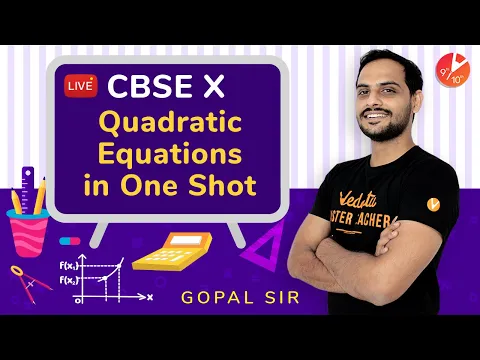Quadratic Equations In one Shot | CBSE Class 10 Maths Chapter 4 | NCERT @VedantuClass910
Vedantu 9&10
5.4K likes
173.3K Views
3 years ago

## NCERT Solutions for Class 10 Maths Chapter 4 Quadratic Equations in Hindi

प्रश्नावली 4.1

1. एक नोटबुक की कीमत एक कलम की कीमत से दो गुनी है। इसकथन को निरूपित करने के लिए दो चरों वाला एक रैखिक समीकरण लिखिए।

(संकेत मान लीजिए, नोटबुक की कीमत $\text{x}$ रु है और कलम की कीमत $\text{y}$ रु हो)

उत्तर: मान लीजिये कि नोटबुक की कीमत $\text{x}$ रु है,

कलम कि कीमत $\text{y}$ रु है I

दिया गया है कि,

नोटबुक कि कीमत = $\text{2}$ (कलम कि कीमत)

$\text{x }=\text{2y}$

$\text{x}-\text{2y }=0$

2. निम्नलिखित रैखिक समीकरणों को $\text{ax }+\text{ by }+\text{ c }=\text{ }0$ के रूप मेंव्यक्त कीजिए और प्रत्येक स्थिति में $\text{a}, \text{ b}$ और $\text{c}$ के मान बताइए:

1. $\mathbf{\text{2x}+\text{3y}=\text{9}.\text{35}}$

उत्तर: $\text{2x}+\text{3y }=\text{9}.\text{35}$

दिए गए समीकरण को $\text{ax}+\text{by}+\text{c}=0$ के रूप में व्यक्त करने पर,

$\text{2x}+\text{3y}-\text{9}.\text{35}=0$

$\text{a }=\text{2}, \text{ b}=\text{3}, \text{ c}=-\text{9}.\text{35}$

1. $\mathbf{\text{x-}\frac{y}{5}-10=0}$

उत्तर: $x-\frac{y}{5}5-10=0$

दिए गए समीकरण को $\text{ax}+\text{by}+\text{c}=0$ के रूप में व्यक्त करने पर,

$x-\frac{y}{5}5-10=0$

$a\text{ }=\text{ }1, \text{ }b\text{ }=\frac{-1}{5}, \text{ }c=-10$

1. $\mathbf{-\text{2x}+\text{3y }=\text{6}}$

उत्तर: $-\text{2x}+\text{3y }=\text{6}$

दिए गए समीकरण को $\text{ax}+\text{by}+\text{c}=0$ के रूप में व्यक्त करने पर,

$-\text{2x}+\text{3y}-\text{6 }=0$

$\text{a}=-\text{2}, \text{ b}=\text{3}, \text{ c}=-\text{6}$

1. $\mathbf{\text{x}=\text{3y}}$

उत्तर: $\text{x}=\text{3y}$

दिए गए समीकरण को $\text{ax}+\text{by}+\text{c}=0$ के रूप में व्यक्त करने पर,

$\text{x}-\text{3y}=0$

$\text{a}=\text{1}, \text{ b}=-\text{3}, \text{ c}=0$

1. $\mathbf{\text{2x}=-\text{5y}}$

उत्तर: $\text{2x}=-\text{5y}$

दिए गए समीकरण को $\text{ax}+\text{by}+\text{c}=0$ के रूप में व्यक्त करने पर,

$\text{2x}+\text{5y}=0$

$\text{a}=\text{2}, \text{ b}=\text{5}, \text{ c}=0$

1. $\mathbf{\text{3x}+\text{2}=0}$

उत्तर: $\text{3x}+\text{2}=0$

दिए गए समीकरण को $\text{ax}+\text{by}+\text{c}=0$ के रूप में व्यक्त करने पर,

$\text{3x}+\text{2}=0$

$\text{a}=\text{3}, \text{ b}=0, \text{c}=\text{2}$

1. $\mathbf{\text{y}-\text{2 }=0}$

उत्तर: y-2 =0

दिए गए समीकरण को $\text{ax}+\text{by}+\text{c}=0$ के रूप में व्यक्त करने पर,

$\text{y}-\text{2}=0$

$\text{a}=0, \text{ b}=\text{1}, \text{c}=-\text{2}$

1. $\mathbf{\text{5}=\text{2x}}$

उत्तर: 5=2x

दिए गए समीकरण को $\text{ax}+\text{by}+\text{c}=0$ के रूप में व्यक्त करने पर,

$\text{2x}-\text{5}=0$

$\text{2x}+0\text{y}-\text{5}=0;\text{ a}=\text{2}, \text{ b}=0, \text{ c}=-\text{5}$

### 1. निम्नलिखित विकल्पों में कौन-सा विकल्प सत्य है, और क्यों?  $\mathbf{\text{y}=\text{3x}+\text{5}}$ का

1. एक द्वितीय हल है

2. केवल दो हल हैं

3. अपरिमित रूप से अनेक हल हैं

उत्तर: (iii) अपरिमित रूप से अनेक हल हैं, क्योंकि दिए गए समीकरण $\text{ax}+\text{by}+\text{c}=0$ रूप में हैं I

2. निम्नलिखित समीकरणों में से प्रत्येक समीकरण के चार हल लिखिए

1. 2x+y=7

उत्तर: $\text{2x}+\text{y}=\text{7}$

$\text{2x}=\text{7}-\text{y}$

$x=\frac{\text{ }7-2}{2}$

जब $\text{y}=0$ ,

तब $x=\frac{\text{ }7-0}{2}$

$\text{x}=$ $\frac{7}{2}$

जब $\text{y}=1$

तब  x = $\frac{7-1}{2}$

$\text{x}=$ $\frac{6}{2}=3$

जब $\text{y}=$ $-\text{1}$

तब $\text{x}=$ $\frac{7+1}{2}$

$\text{x}=$ $\frac{8}{2}=4$

जब $\text{y}=\text{7}$

तब $\text{x}=$ $\frac{7-7}{2}=2$

$\text{x}=0$

अत: $\text{x}$ और $\text{y}$ का दिए गए समीकरण के लिए चार हलनिम्नलिखित है:

 $\text{x}$ $\frac{7}{2}$ $3$ $4$ $0$ $\text{y}$ $0$ $1$ $-1$ $7$
1. 𝜋 $\mathbf{\text{x}+\text{y}=\text{9}}$

उत्तर: 𝜋 $\text{x}+\text{y}=\text{9}$

𝜋 $\text{x}=\text{9}-\text{y}$

$x=\text{ }\frac{\text{9-y}}{\pi }$

जब $\text{y }=\text{1}$

तब $x=\text{ }\frac{9-1}{\pi }$

$\text{x}=$ $\frac{8}{\pi }$

जब $\text{y }=\text{2}$

तब $x=\text{ }\frac{9-2}{\pi }$

$x=\text{ }\frac{7}{\pi }$

जब $\text{y }=-\text{1}$

तब $x=\text{ }\frac{9+1}{\pi }$

$x=\text{ }\frac{10}{\pi }$

जब $\text{y }=0$

तब $x=\text{ }\frac{9-0}{\pi }$

$x=\text{ }\frac{9}{\pi }$

अत: $\text{x}$ और $\text{y}$ का दिए गए समीकरण के लिए चार हलनिम्नलिखित है:

 $\text{x}$ $\frac{8}{\pi }$ $\frac{7}{\pi }$ $\frac{10}{\pi }$ $\frac{9}{\pi }$ $\text{y}$ $1$ $2$ $-1$ $0$
1. $\mathbf{x=4y}$

उत्तर: $x=4y$

जब $\text{y}=0$

तब $\text{x}=\text{4}\left( 0 \right)$

$\text{x}=0$

जब $\text{y}=\text{1}$

तब $\text{x}=\text{4}\left( \text{1} \right)$

$\text{x}=\text{4}$

जब $\text{y}=\text{2}$

तब $\text{x}=\text{4}\left( \text{2} \right)$

$\text{x}=\text{8}$

जब $\text{y}=\text{3}$

तब $\text{x}=\text{4}\left( \text{3} \right)$

$\text{x}=\text{12}$

अत: $\text{x}$ और $\text{y}$ का दिए गए समीकरण के लिए चार हलनिम्नलिखित है:

 $\text{x}$ $0$ $\text{4}$ $\text{8}$ $\text{12}$ $\text{y}$ $0$ $\text{1}$ $\text{2}$ $\text{3}$

3. बताइए कि निम्नलिखित हलों में कौन-कौन समीकरण x - 2y = 4 के हल है और कौन-कौन नहीं है:

उत्तर: दिए गए समीकरण $\mathbf{\text{x}-\text{2y}=\text{4}}$

1. $\mathbf{\left( 0, \text{2} \right)}$

उत्तर: $\left( 0, \text{2} \right)$

जब $\text{x}=0, \text{ y}=\text{2}$

तब $\text{x}-\text{2y}=\text{4}$

$0-\text{2}\left( \text{2} \right)\text{ }=\text{4}$

$-\text{4}\ne \text{4}$

अतः $\left( 0, \text{2} \right), \text{ x}-\text{2y}=0$ समीकरण का हल नहीं है I

1. $\mathbf{\left( \text{2}, 0 \right)}$

उत्तर: $\left( \text{2}, 0 \right)$

जब $\text{x}=\text{2}, \text{ y}=0$

तब $\text{x}-\text{2y}=\text{4}$

$\text{2}-\text{2}\left( 0 \right)\text{ }=\text{4}$

$\text{2}\ne \text{4}$

अतः $\left( \text{2}, 0 \right), \text{ x}-\text{2y}=0$ समीकरण का हल नहीं है I

1. $\mathbf{\left( \text{4}, 0 \right)}$

उत्तर: $\left( \text{4}, 0 \right)$

जब $\text{x}=\text{4}, \text{ y}=0$

तब $\text{x}-\text{2y}=\text{4}$

$\text{4}-\text{2}\left( 0 \right)\text{ }=\text{4}$

$\text{4}=\text{4}$

अतः $\left( \text{4}, 0 \right), \text{ x}-\text{2y}=0$ समीकरण का हल हैI

1. $\mathbf{\left( \surd \text{2}, \text{ 4}\surd \text{2} \right)}$

उत्तर: $\left( \surd \text{2}, \text{ 4}\surd \text{2} \right)$

जब $\text{x}=\surd \text{2}, \text{ y}=\text{4}\surd \text{2}$

तब $\text{x}-\text{2y}=\text{4}$

$\surd \text{2}-\text{2}\left( \text{4}\surd \text{2} \right)\text{ }=\text{4}$

$\surd \text{2}-\text{8}\surd \text{2}$

$-\text{7}\surd \text{2 }\ne \text{ 4}$

अतः $(\surd \text{2}, \text{ 4}\surd \text{2}), \text{ x}-\text{2y}=0$ समीकरण का हल नहीं है I

1. $\mathbf{\left( \text{1}, \text{1} \right)}$

उत्तर: $\left( \text{1}, \text{1} \right)$

जब $\text{x}=\text{1}, \text{ y}=\text{1}$

तब $\text{x}-\text{2y}=\text{4}$

$\text{1}-\text{2}\left( \text{1} \right)\text{ }=\text{4}$

$-\text{1 }\ne \text{4}$

अतः $\left( \text{1}, \text{1} \right), \text{ x}-\text{2y}=0$ समीकरण का हल नहीं है I

4. k का मान ज्ञात कीजिए जबकि $\mathbf{\text{x }=\text{2}, \text{ y }=\text{1}}$ समीकरण $\mathbf{\text{2x }+\text{ 3y }=\text{k}}$ का एक हल हो I

उत्तर: $\text{2x }+\text{ 3y }=\text{k}$

जब $\text{x}=\text{2}, \text{ y}=\text{1}$

तब, $\text{2}\left( \text{2} \right)\text{ }+\text{3}\left( \text{1} \right)\text{ }=\text{k}$

$\text{4}+\text{3}=\text{k}$

$\text{k }=\text{7}$

### प्रश्नावली 4.3

1. दो चरों वाले निम्नलिखित रैखिक समीकरणों में से प्रत्येक काआलेख खींचिए:

1. $\mathbf{\text{x}+\text{y}=\text{4}}$

उत्तर: $\text{x}+\text{y}=\text{4}$

$\text{x}=\text{4}-\text{y}$

 $\text{x}$ $0$ $\text{1}$ $\text{2}$ $\text{y}$ $\text{4}$ $\text{3}$ $\text{2}$ .

बिन्दुओं $(0, \text{4})\text{ }\left( \text{1}, \text{3} \right)\text{ }\left( \text{2}, \text{2} \right)$ का आलेख है: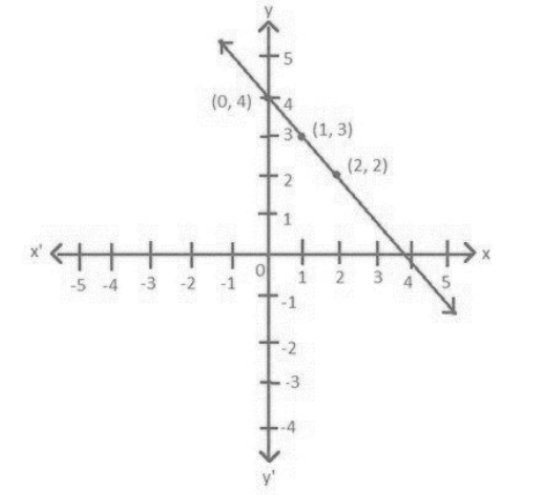1. $\mathbf{\text{x}-\text{y}=\text{2}}$

उत्तर: $\text{x}-\text{y}=\text{2}$

$\text{x}=\text{y}+\text{2}$

 $\text{x}$ $\text{3}$ $\text{4}$ $\text{5}$ $\text{y}$ $\text{1}$ $\text{2}$ $\text{3}$ .

बिन्दुओं $\left( \text{3}, \text{1} \right)\text{ }\left( \text{4}, \text{2} \right)\text{ }\left( \text{5}, \text{3} \right)$ का आलेख है: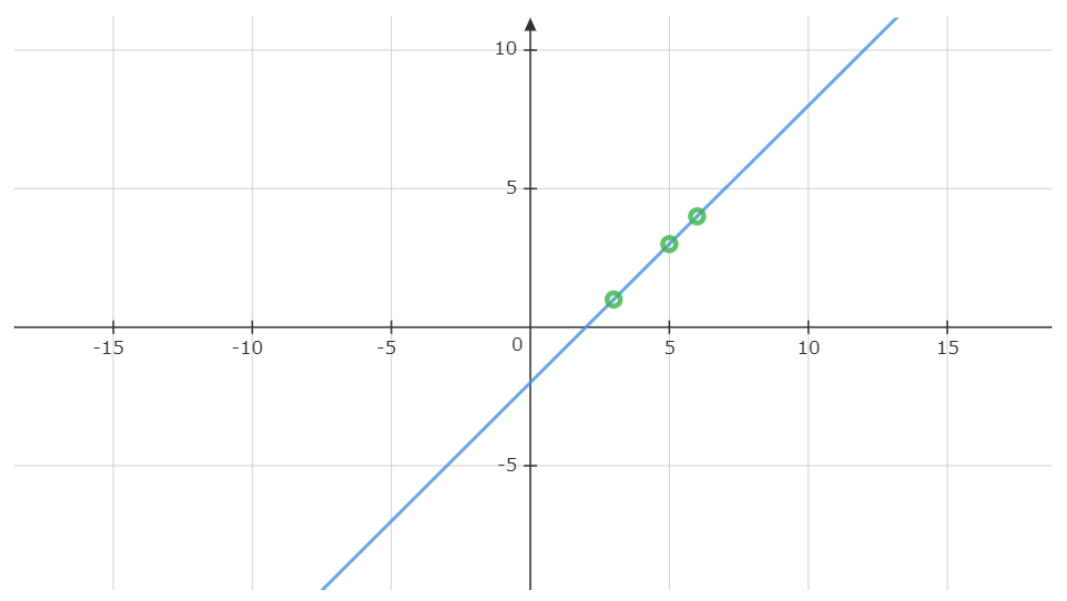1. $\mathbf{\text{y}=\text{3x}}$

उत्तर: $\text{y}=\text{3x}$

 $\text{x}$ $0$ $\text{1}$ $-\text{1}$ $\text{y}$ $0$ $\text{3}$ $-\text{3}$ .

बिन्दुओं (0, 0) (1, 3) (-1, -3) का आलेख है: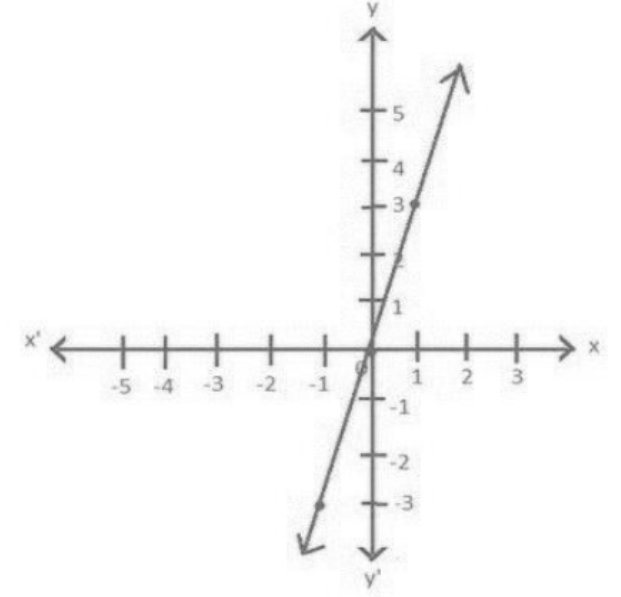1. $\mathbf{\text{3}=\text{2x}+\text{y}}$

उत्तर: $\text{3}=\text{2x}+\text{y}$

$\text{y }=$ $\text{3}-\text{2x}$

 $\text{x}$ $0$ $\text{1}$ $-\text{1}$ $\text{y}$ $\text{3}$ $\text{1}$ $-\text{5}$ .

बिन्दुओं $\left( 0, \text{3} \right)\text{ }\left( \text{1}, \text{1} \right)\text{ }\left( -\text{1}, -\text{5} \right)$ का आलेख है: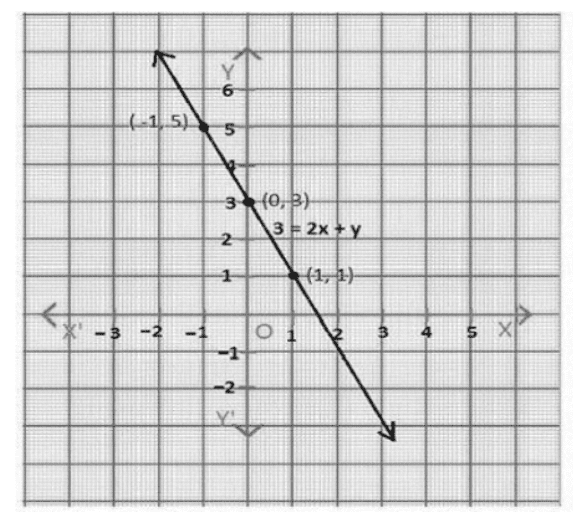2. बिंदु $\mathbf{\left( \text{2}, \text{ 14} \right)}$ से होकर जाने वाली दो रेखाओं के समीकरण लिखिए | इसप्रकार की और कितनी रेखाएँ हो सकती है, और क्यों ?

उत्तर: दिए गए बिंदु में $\left( \text{2}, \text{14} \right)$ में $\text{x}=\text{2}, \text{y}=\text{14}$

इस मान को संतुष्ट करने वाले दो समीकरण निम्न हैं:

$\text{x}+\text{y}=\text{16}$

$\text{x}-\text{y}=-\text{12}$

इस प्रकार बहुत सारे रेखा इस बिंदु $\left( \text{2}, \text{14} \right)$ से गुजरती है I

3. यदि बिंदु $\mathbf{\left( \text{3}, \text{ 4} \right)}$ समीकरण $\mathbf{\text{3y }=\text{ax }+\text{ 7}}$ के आलेख पर स्थितहै, तो a का मान ज्ञात कीजिए |

उत्तर: $\text{3y }=\text{ax }+\text{ 7}$

$\left( \text{3}, \text{4} \right)$ में $\text{x}=\text{3}$ और $\text{y}=\text{4}$ , समीकरण $\text{3y}=\text{ax}+\text{7}$ में $\text{x}$ और $\text{y}$ का मान रखने पर

$\text{3}\left( \text{4} \right)=\text{3a }+\text{7}$

$\text{12}=\text{3a}+\text{7}$

$\text{3a}=\text{12}-\text{7}$

$\text{3a}=\text{5}$

4. एक नगर में टैक्सी का किराया निम्नलिखित है: पहले किलोमीटर का किराया $\text{8}$ रु है और उसके बाद की दूरी के लिए प्रति किलोमीटर का किराया $\text{5}$ रु है। यदि तय की गई दूरी $\text{x}$ किलोमीटर हो, और कुल किराया $\text{y}$ रु हो, तो इसका एक रैखिक समीकरणलिखिए और उसका आलेख खींचिए।

उत्तर: तय की हुआ दुरी = $\text{x}$ km

कुल किराया = $\text{y}$ रु

यह दिया गया है कि,

पहले किलोमीटर का किराया + $\text{5}$ (तय किये गई दूरी- $\text{1}$ )= $\text{y}$

$\text{8 }+\text{ 5}\left( \text{x }\text{ 1} \right)\text{ }=\text{y}$

$\text{8 }+\text{ 5x }\text{ 5 }=\text{y}$

$\text{3 }+\text{ 5x }=\text{y}$

$\text{5x }\text{y }+\text{ 3 }=0$

$\text{y }=\text{5x }+\text{ 3}$

 $\text{x}$ $0$ $-\text{1}$ $\text{1}$ $\text{y}$ $\text{3}$ $-\text{2}$ $\text{8}$ .

बिन्दुओं $\left( 0, \text{3} \right)\text{ }\left( -\text{1}, -\text{2} \right)\text{ }\left( \text{1}, \text{8} \right)$ का आलेख है: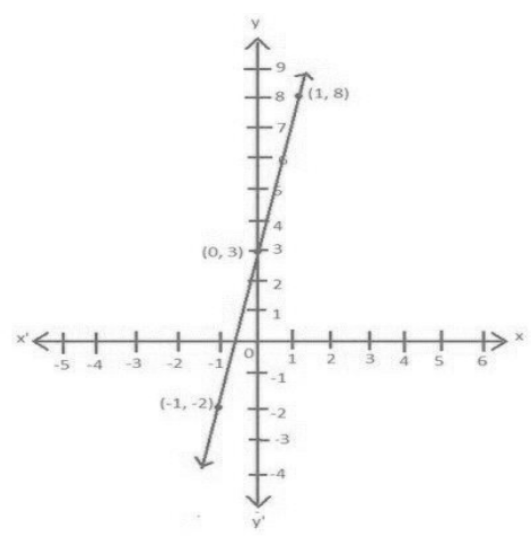5. निम्नलिखित आलेखों में से प्रत्येक के लिए दिए गए विकल्पों से सही समीकरण का चयन कीजिए: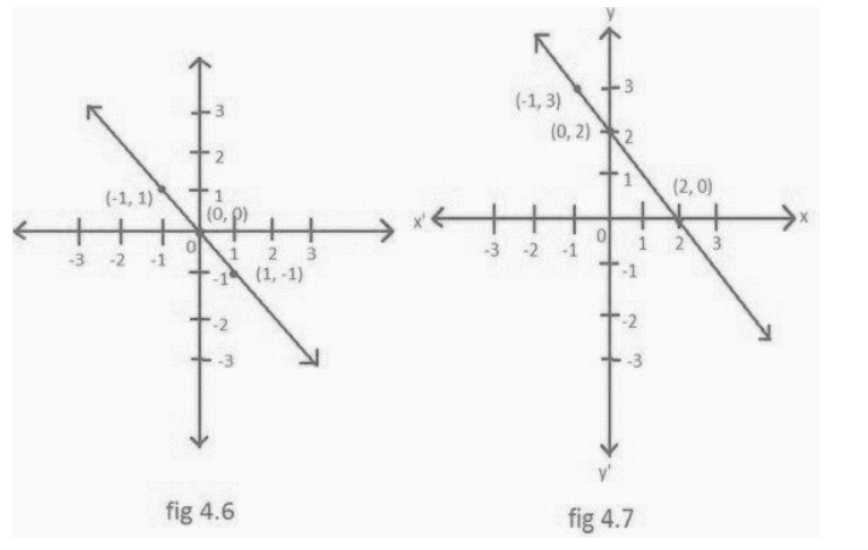आकृति 4.6 के लिए

1. $\text{y}=\text{x}$

2. $\text{x}+\text{y}=0$

3. $\text{y}=\text{2x}$

4. $\text{2}+\text{3y}=\text{7x}$

उत्तर: आकृति 4.6 के लिए

ii) $\text{x}+\text{y}=0$

आकृति 4.7 के लिए

i. $\text{y}=\text{x}+\text{2}$

ii. $\text{y}=\text{x}-\text{2}$

iii. $\text{y}=-\text{x}+\text{2}$

iv. $\text{x}+\text{2y}=\text{6}$

उत्तर: आकृति 4.7 के लिए

iii) $\text{y}=-\text{x}+\text{2}$

6. एक अचर बल लगाने पर एक पिंड द्वारा किया गया कार्य पिंड द्वारा तय की गई दूरी के अनुक्रमानुपाती होता है। इस कथन को दो चरों वाले एक समीकरण के रूप में व्यक्त कीजिए और अचर बल $\text{5}$ मात्रक लेकर इसका आलेख खींचिए। यदि पिंड द्वारा तय कीगई दूरी

1. $\text{2}$ मात्रक

2. $0$ मात्रक

हो, तो आलेख से किया हुआ कार्य ज्ञात कीजिए।

उत्तर: माना किया गया कार्य = $\text{y}$

पिंड द्वारा विस्थापन = $\text{x}$ मीटर

अचर बल = $\text{5}$ इकाई

किया गया कार्य =बल × विस्थापन

$\text{W }=\text{F }\times \text{ S}$

अतः $\text{y }=\text{5x}$

i. $\text{2}$ मात्रक

जब $\text{x}=\text{2}$ ,

$\text{y}=\text{5}\left( \text{2} \right)\text{ }=\text{1}0$

अतः किया गया कार्य $\text{1}0$ मात्रक है I

ii. $0$ मात्रक

जब $\text{x}=0$ ,

$\text{y}=\text{5}\left( 0 \right)=0$

अतः किया गया कार्य $0$ मात्रक है I

 $x$ $-1$ $0$ $1$ $y$ $-5$ $0$ $5$

बिन्दुओं $(-\text{1}, -\text{5})\text{ }\left( 0, 0 \right)\text{ }\left( \text{1}, \text{5} \right)$ का आलेख है: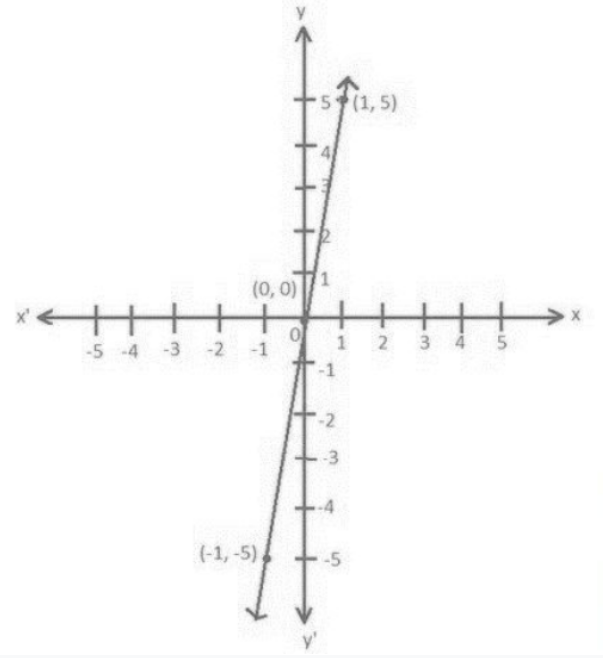7. एक विद्यालय की कक्षा $\text{IX}$ की छात्राएं यानमनी और फातिमा नेमिलकर भूकंप पीड़ित व्यक्तियों की सहायता के लिए प्रधानमंत्री राहत कोष में $\text{1}00$ रु अंशदान दिया। एक रैखिक समीकरण लिखिए जो इनआंकड़ो को संतुष्ट करती हो। (आप उनका अंशदान रु $\text{x}$ और रु $\text{y}$ मान सकते हैं। इस समीकरण का आलेख खींचिए।

उत्तर: उत्तर: मानना है कि यामिनी द्वारा योगदान = $\text{x}$ रु

और फातिमा द्वारा योगदान = $\text{y}$ रु

दोनों के द्वारा दिया गया अंशदान = $\text{1}00$ रु

अत:

$\text{x }+\text{ y }=\text{1}0$

y =100 - x

 $\text{x}$ $\text{1}0$ $20$ $30$ $y$ $90$ $80$ $70$ .

बिन्दुओं $\left( \text{1}0, \text{9}0 \right)\text{ }\left( \text{2}0, \text{8}0 \right)\text{ }\left( \text{3}0, \text{7}0 \right)$ का आलेख है: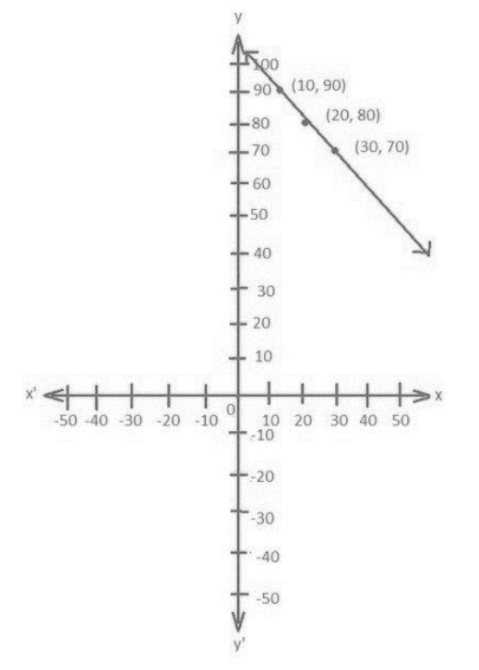8. अमरीका और कनाडा जैसे देशों में तापमान फारेनहाइट मेंमापा जाता है, जबकि भारत जैसे देशों में तापमान सेल्सियस में मापा जाता है। यहाँ फारेनहाइट को सेल्सियस में रूपांतरित करने वाला एकरैखिक समीकरण दिया गया है:

$F=\left( \text{ }\frac{9\text{ }}{5} \right)C+32$

(i) सेल्सियस को x-अक्ष और फारेनहाइट को y-अक्ष मानकर ऊपर दिए गए रैखिक समीकरण का आलेख खींचिए।

उत्तर: $F=(\frac{9}{5})C+32$

मन फारेनहाईट $\text{F}=\text{y}$

सेल्सियस $\text{C}=\text{x}$

तब $y=(\frac{9}{5})x+32$

जब $\text{x}=\text{1}0$

$y=(\frac{9}{5})(10)+32$

$\text{y}=\text{5}0$

 $\text{x}$ $\text{1}0$ $20$ $30$ $\text{y}$ $50$ $68$ $86$ .

बिन्दुओं $\left( \text{1}0, \text{5}0 \right)\text{ }\left( \text{2}0, \text{68} \right)\text{ }\left( \text{3}0, \text{86} \right)$ का आलेख है: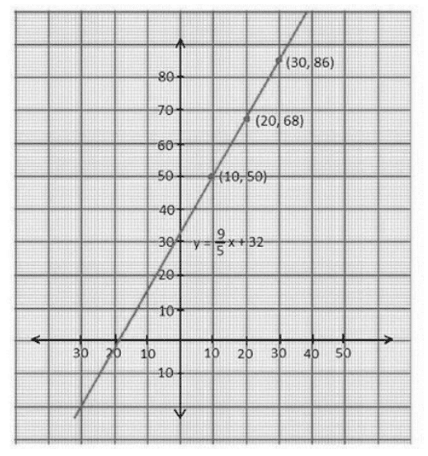(ii) यदि तापमान $\text{3}0{}^\circ \text{C}$ है, तो फारेनहाइट में तापमान क्या होगा?

उत्तर: $\text{C}$ का मान $\text{3}0{}^\circ$ रखने पर

$F=(\frac{9}{5})(30)+32$

$\text{F}=\text{54}+\text{32}$

$\text{F}=\text{86}$

(iii) यदि तापमान $\text{95}{}^\circ \text{F}$ है, तो सेल्सियस में तापमान क्या होगा?

उत्तर: $\text{F}$ का तापमान $\text{95}{}^\circ$ रखने पर

$F=(\frac{9}{5})C+32$

$\text{95=(}\frac{9}{5}\text{)C+32}$

$\text{(}\frac{9}{5}\text{)C=95-32}$

$\text{(}\frac{9}{5}\text{)C=63}$

$\text{C=}\frac{63\times 5}{9}$

$\text{C}=\text{35}$

(iv) यदि तापमान $0{}^\circ \text{C}$ है, तो फारेनहाइट में तापमान क्या होगा? और यदि तापमान $0{}^\circ \text{F}$ है, तो सेल्सियस में तापमान क्या होगा?

उत्तर: $\text{C}$ का तापमान $0{}^\circ$ रखने पर.

$F=(\frac{9}{5})(0)+32$

$\text{F}=\text{32}$

(v) क्या ऐसा भी कोई तापमान है जो फारेनहाइट और सेल्सियस दोनों के लिए संख्यात्मक समान है? यदि हाँ, तो उसे ज्ञात कीजिए।

उत्तर: जब $\text{F}$ और $\text{C}$ का संख्यात्मक रूप एक सामान है,

$\text{F}=\text{C}=\text{t}$

$F=(\frac{9}{5})C+32$

$t=(\frac{9}{5})t+32$

$\text{5t}=\text{9t}+\text{16}0$

$\text{5t}-\text{9t}=\text{16}0$

$-\text{4t}=\text{16}0\text{ t}=-\text{4}0$

### प्रश्नावली 4.4

1. (i) एक चर वाले

(ii) दो चर वाले

समीकरण के रूप में $\text{y }=\text{3}$ का ज्यामितिय निरूपण कीजिए।

उत्तर :

i. एक चर वाले समीकरण के रूप में $\text{y }=\text{3}$ का ज्यामितिय निरूपण: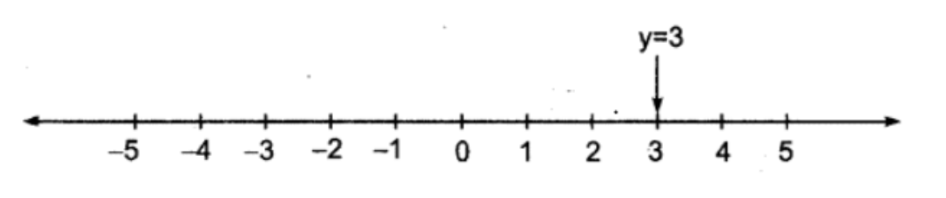अतः $\text{y }=\text{3}$ की संख्या - रेखा पर यही ज्यामितिय स्थिति है।

ii. दो चर वाले समीकरण के रूप में $\text{y }=\text{3}$ को ज्यामितिय निरूपण:

1. (वर्ग पत्रक) ग्राफ पेपर पर $X-$ अक्ष तथा $\text{Y}-$ अक्ष खींचकर उन पर मापन चिह्न अंकित कीजिए।

2. $\text{Y}-$ अक्ष पर $+\text{3}$ चिह्न से $X-$ अक्ष के समान्तर रेखा $\text{AB}$ खींचिए।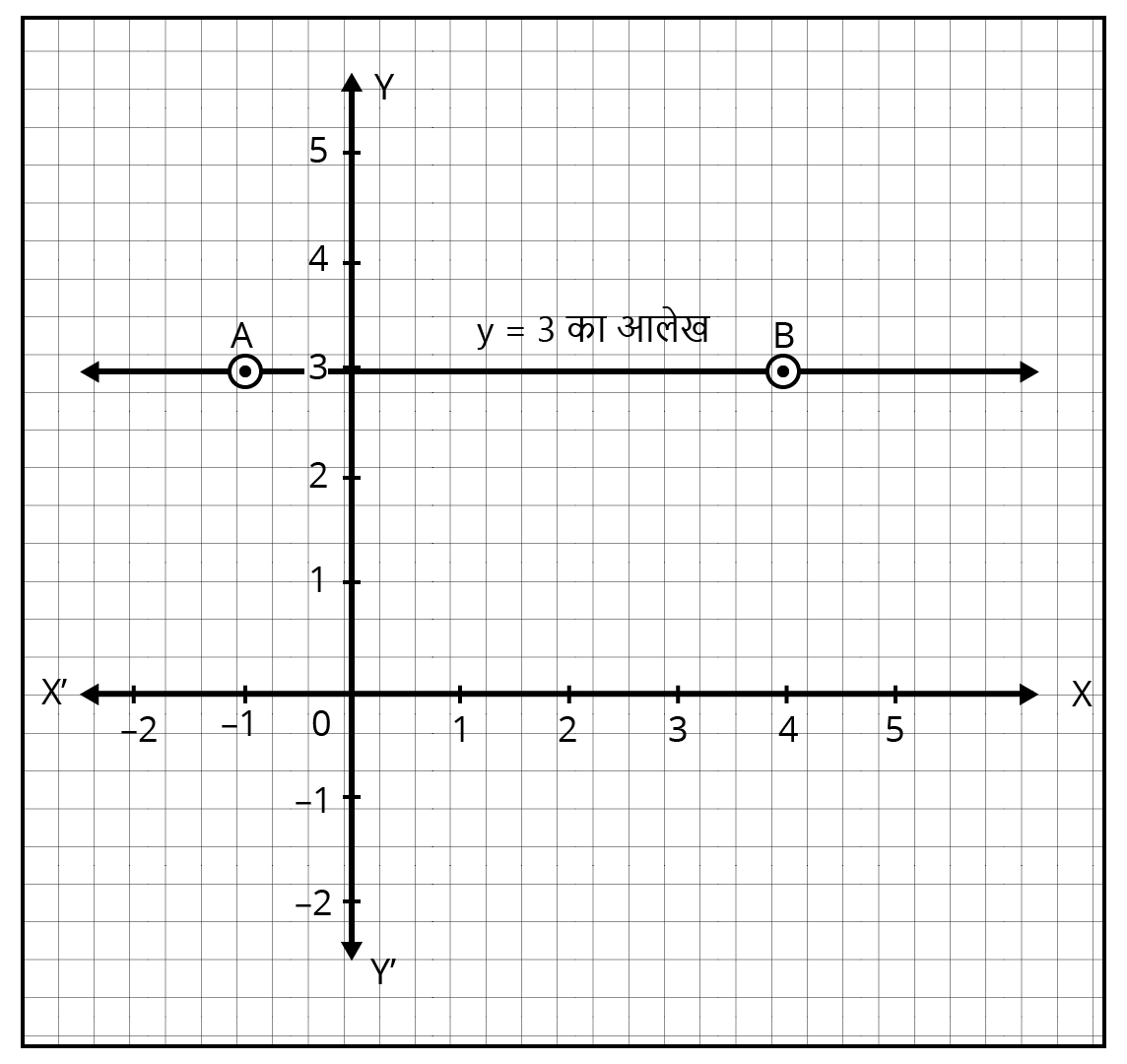2. (i) एक चर वाले

(ii) दो चर वाले

समीकरण के रूप में $\text{2x }+\text{ 9 }=0$ का ज्यामितिय निरूपण कीजिए।

उत्तर :

i. एक चर वाले समीकरण के रूप में $\text{2x }+\text{ 9 }=0$ का ज्यामितिय निरूपण:

$\text{2x }+\text{ 9 }=0$

$\text{2x }=-\text{9}$

$\text{x }=-\text{4}.\text{5}$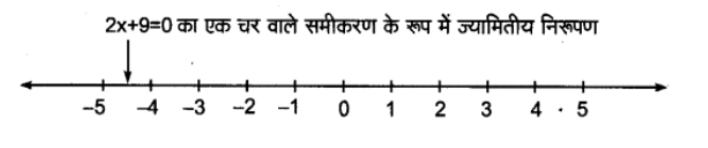ii. दो चार वाले समीकरण के रूप में $\text{2x }+\text{ 9 }=0$ का ज्यामितीय निरूपण:

1. ग्राफ पेपर पर $\text{X}-$ अक्ष तथा $\text{Y}-$ अक्ष खींचकर उन पर मापक चिन्ह अंकित कीजिए।

2. $\text{X}-$ अक्ष पर $\frac{-9}{2}$ या $-4.5$ चिन्हितअंकित कीजिए और इससे $\text{Y}-$ अक्ष के समान्तर रेखा $\text{AB}$ खींचिए।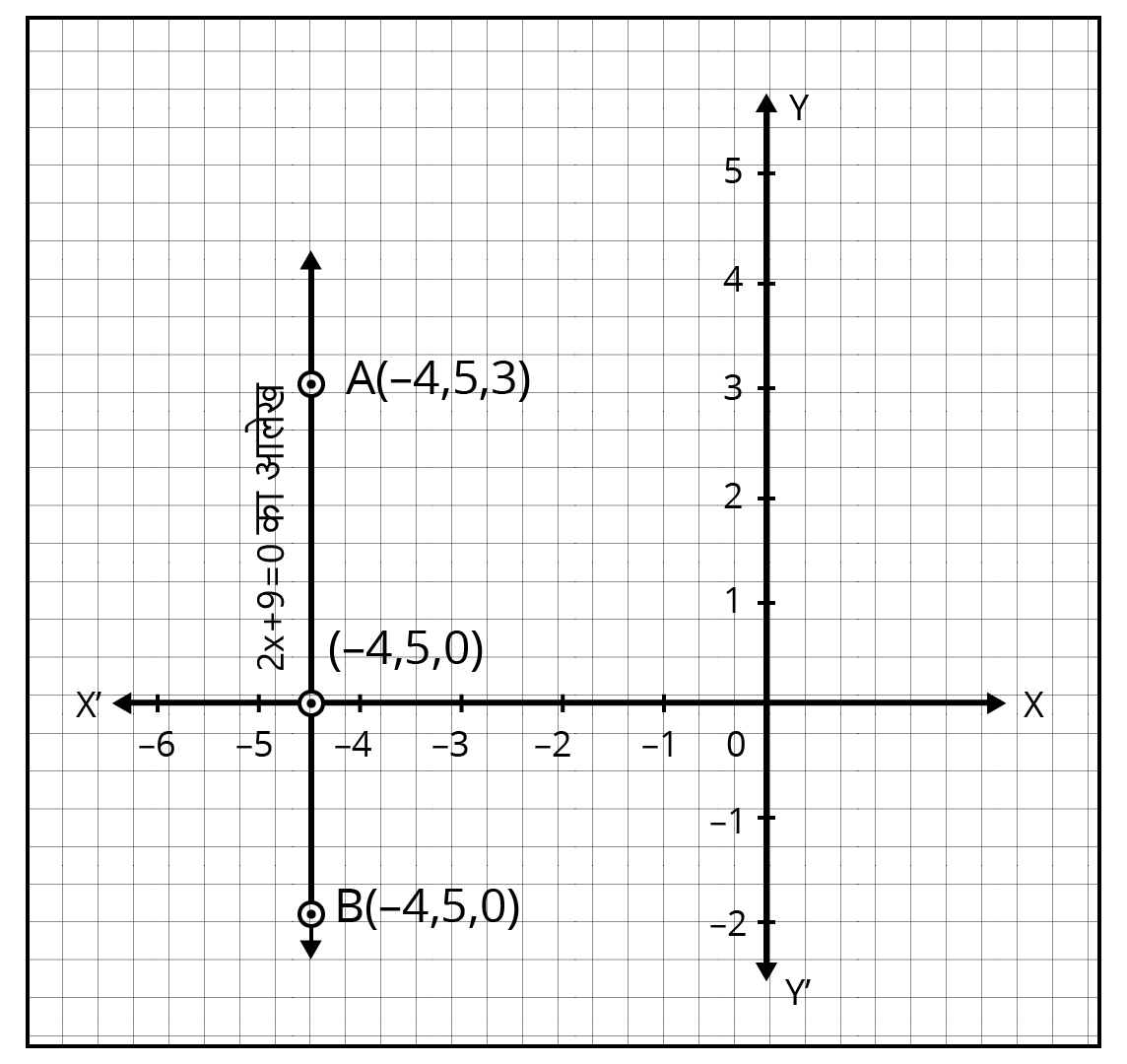### NCERT Solutions for Class 10 Maths Chapter 4 Quadratic Equations in Hindi

Chapter-wise NCERT Solutions are provided everywhere on the internet with an aim to help the students to gain a comprehensive understanding. Class 10 Maths Chapter 4 solution Hindi mediums are created by our in-house experts keeping the understanding ability of all types of candidates in mind. NCERT textbooks and solutions are built to give a strong foundation to every concept. These NCERT Solutions for Class 10 Maths Chapter 4 in Hindi ensure a smooth understanding of all the concepts including the advanced concepts covered in the textbook.

NCERT Solutions in Hindi medium have been created keeping those students in mind who are studying in a Hindi medium school. These NCERT Solutions for Class 10 Maths Quadratic Equations in Hindi medium pdf download have innumerable benefits as these are created in simple and easy-to-understand language. The best feature of these solutions is a free download option. Students of Class 10 can download these solutions at any time as per their convenience for self-study purpose.

These solutions are nothing but a compilation of all the answers to the questions of the textbook exercises. The answers/ solutions are given in a stepwise format and very well researched by the subject matter experts who have relevant experience in this field. Relevant diagrams, graphs, illustrations are provided along with the answers wherever required. In nutshell, NCERT Solutions for Class 10 Maths in Hindi come really handy in exam preparation and quick revision as well prior to the final examinations.

## FAQs on NCERT Solutions for Class 10 Maths Chapter 4 - In Hindi

1. How can I get good marks in Class 10 Maths Chapter 4 Quadratic Equations?

It is easy to get good scores in  Chapter 4 Quadratic Equations portion in the Class 10 Maths examination. All you need to do is practice the NCERT examples and exercise questions diligently and then refer to other study materials. The best guide that will help you in your practice is Vedantu’s NCERT Solutions for Class 10 Maths Chapter 4. Students can also avail the NCERT Solutions Class 10 Maths Chapter 4 in Hindi on the Vedantu app. All the material is free of cost.

2. How is a quadratic polynomial different from a quadratic equation in Class 10 Maths Chapter 4?

A quadratic polynomial is a simple polynomial that consists of variables and coefficients with the greatest degree two. It can be expressed as ax2 + bx + c. There is no equal sign. In a quadratic equation, a quadratic polynomial with a degree of 2 is expressed in equation form, where ax2 + bx + c = 0. In a quadratic equation, the polynomial function with a power of two is equated to zero.

3. How many exercises are there in NCERT Solutions for Class 10 Maths Chapter 4?

There are four exercises in the NCERT Class 10 Maths Chapter 4 “Quadratic Equations”. All these exercises are very important from the exam point of view and must be practiced to get a good hold over the various concepts mentioned in the chapter. If you follow the examples carefully, you will not have difficulty in solving the questions. For simplified clarifications in Hindi, you can refer to NCERT Solutions Class 10 Maths Chapter 4 in Hindi available on the official website of  Vedantu.

4. What is the standard form of quadratic equation in Class 10 Maths Ex. 4.1?

The standard form of a quadratic equation is ax2 + bx + c = 0, where a, b, and c represents a real number. The greatest degree in a quadratic equation is two. A standard quadratic equation can be solved by the factorisation method or by solving the square. To know more about the solutions of exercise 4.1 and other exercises of the chapter, you can use Vedantu’s NCERT Solutions. NCERT Solutions Class 10 Maths Chapter 4 in Hindi is also conveniently available.

5. In Class 10 Maths Chapter 4, which exercise is considered the most difficult to solve?

All exercises in the NCERT Class 10 Maths Chapter 4 are equally important. The level of difficulty of these exercises depends on the preparation of the student. If you practice examples and exercises thoroughly, you will be able to solve all questions. For the best preparation, you can use the NCERT Solutions available on Vedantu for detailed answers.